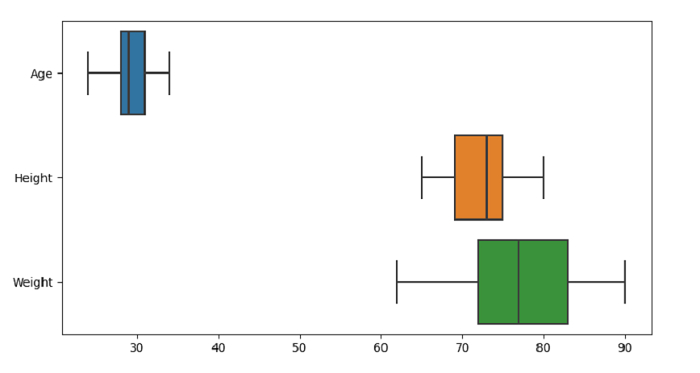# Python Pandas - Draw a boxplot for each numeric variable in a DataFrame with Seaborn

Box Plot in Seaborn is used to draw a box plot to show distributions with respect to categories. The seaborn.boxplot() is used for this. Use the "orient” parameter for orientation of each numeric variable.

Let’s say the following is our dataset in the form of a CSV file − Cricketers.csv

At first, import the required libraries −

import seaborn as sb
import pandas as pd
import matplotlib.pyplot as plt

Load data from a CSV file into a Pandas DataFrame −

dataFrame = pd.read_csv("C:\Users\amit_\Desktop\Cricketers.csv")

Plotting box plot using the orient parameter for orientation of each numeric variable −

sb.boxplot( data = dataFrame, orient="h")

## Example

Following is the code −

import seaborn as sb
import pandas as pd
import matplotlib.pyplot as plt

# Load data from a CSV file into a Pandas DataFrame:

# plotting box plot
# using the orient parameter for orientation of each numeric variable
sb.boxplot( data = dataFrame, orient="h")

# display
plt.show()

## Output

This will produce the following output −